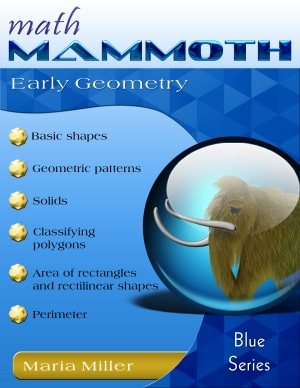You are here: HomeOnline resourcesGeometry → Area & perimeter

# Online games, worksheets, and activities for area & perimeter

This is an annotated and hand-picked list of online games, worksheets, tutorials and activities for area and perimeter, suiting best grades 3-6.

## Area and perimeter

Free worksheets for the area & perimeter of rectangles
An unlimited supply of worksheets for the area and perimeter of rectangles/squares. Includes worksheets for finding the area, perimeter, or the missing side, word problems, writing an expression for the area of a two-part rectangle, complex rectangular areas, and more. Available both in PDF and html formats.
/worksheets/area_perimeter_rectangles.php

Free worksheets for the area of triangles, quadrilaterals, and polygons
Find the area of triangles, parallelograms, other quadrilaterals, and polygons (pentagons/hexagons) in the coordinate grid.
/worksheets/area_triangles_polygons.php

Everything you wanted to know about area and perimeter
Short explanations of how to find the perimeter of simple shapes and the area of rectangles, followed by quizzes on three levels. In perimeter, level two, some side lengths are not given. In level three, you calculate the perimeter of compound shapes. In area of rectangles, level 1 has just rectangles, and levels 2 and 3 have compound shapes made of rectangles.
www.bgfl.org/custom/resources_ftp/client_ftp/ks2/maths/perimeter_and_area/index.htmlMath Mammoth Early Geometry
A self-teaching worktext for 1st - 3rd grades that covers basic shapes, right angle, symmetry, area, perimeter, and solids. Available both as a download (\$5.95) and as a printed copy.
www.mathmammoth.com/early_geometry.php

Shape Explorer
Find the perimeter and area of odd shapes on rectangular grid.
www.shodor.org/interactivate/activities/ShapeExplorer

Math Playground: Measuring the Area and Perimeter of Rectangles
Amy and her brother, Ben, explain how to find the area and perimeter of rectangles and show you how changing the perimeter of a rectangle affects its area. After the lesson, you will use an interactive ruler to measure the length and width of 10 rectangles, and to calculate the perimeter and area of each.
www.mathplayground.com/area_perimeter.html

Math Playground: Party Designer
You need to design areas for the party, such as crafts table, food table, seesaw, and so on, so that they have the given perimeters and areas.
www.mathplayground.com/PartyDesigner/PartyDesigner.html

BBC Bitesize - Perimeter
A simple revision (review) "bite" for perimeter that includes short explanations and a few quiz questions.
www.bbc.co.uk/bitesize/ks3/maths/measures/perimeter/revision/1/

BBC Bitesize - Area
Brief revision (review) "bites", including a few interactive questions, about area: counting squares, area of rectangles, area of triangles, parallelograms, and of compound shapes. Includes an activity and a test.
www.bbc.co.uk/bitesize/ks3/maths/measures/area/revision/1/

Geometry Area/Perimeter Quiz from ThatQuiz.org
An online quiz, asking either the area or perimeter of rectangles, triangles, and circles. You can modify the quiz parameters to your liking, for example to omit the circle, or instead of solving for area, you solve for an unknown side when perimeter/area is given.
www.thatquiz.org/tq-4/?-j201v-lc-m2kc0-na-p0

Perimeter Game from Cyram.org
A simple online quiz for finding the perimeter of rectangles, triangles, or compound rectangles where not all side lengths are given.
www.cyram.org/Projects/perimetergame/index.html

FunBrain: Shape Surveyor Geometry Game
A simple & easy game that practices finding either the perimeter or area of rectangles.
www.funbrain.com/poly/index.htmlDr. Math Introduces Geometry: Learning Geometry is Easy! Just ask Dr. Math!
A supplement to middle school geometry, Dr. Math Introduces Geometry has a light tone, clear layout, and humorous cartoons by Jessica Wolk-Stanley. The best part is that the explanations to math questions are accurate and clear. You'll find answers to dozens of real questions from students who needed help understanding the basic concepts of geometry. This book covers angles, triangles, quadrilaterals, area, perimeter, Pi, circle parts, polyhedra, surface area, volume, nets, congruent transformations, and symmetry. Price ~\$12 new, ~\$6 used.
www.amazon.com

Area of a Triangle
In this activity, you try to make a parallelogram using a copy of the given triangle.
illuminations.nctm.org/Activity.aspx?id=4160

Area of a Parallelogram
In this activity, you rearrange the pieces in a parallelogram to make a rectangle.
illuminations.nctm.org/Activity.aspx?id=4158

Area Tool
Use this tool to determine how the length of the base and the height of a figure can be used to determine its area. Can you find the similarities and differences between the area formulas for trapezoids, parallelograms, and triangles?
illuminations.nctm.org/Activity.aspx?id=3567

Triangle Explorer
Practice calculating the area of a triangle using this interactive tool.
www.shodor.org/interactivate/activities/TriangleExplorer

#### Key to Geometry Workbooks

Here is a non-intimidating way to prepare students for formal geometry. Key to Geometry workbooks introduce students to a wide range of geometric discoveries as they do step-by-step constructions. Using only a pencil, compass, and straightedge, students begin by drawing lines, bisecting angles, and reproducing segments. Later they do sophisticated constructions involving over a dozen steps-and are prompted to form their own generalizations. When they finish, students will have been introduced to 134 geometric terms and will be ready to tackle formal proofs.

Area of Compound Shapes (MEP book 7)
A tutorial with many interactive exercises about compound rectangular shapes (you can divide them into rectangles).
www.cimt.plymouth.ac.uk/projects/mepres/book7/bk7i9/bk7_9i4.htm

Area of Triangles (MEP book 7)
A tutorial with many interactive exercises about the area of triangle, including compound shapes that with triangles & rectangles.
www.cimt.plymouth.ac.uk/projects/mepres/book7/bk7i9/bk7_9i5.htm

Area of Compound Shapes Quiz
An online multiple-choice quiz about area of basic shapes and area of compound shapes.
www.proprofs.com/quiz-school/story.php?title=area-compound-shapes

XP Math: Find Perimeters of Parallelograms
This online quiz shows you parallelograms and rectangles, and you need to calculate the perimeter, including typing in the right unit, and not using the altitude of the parallelogram.